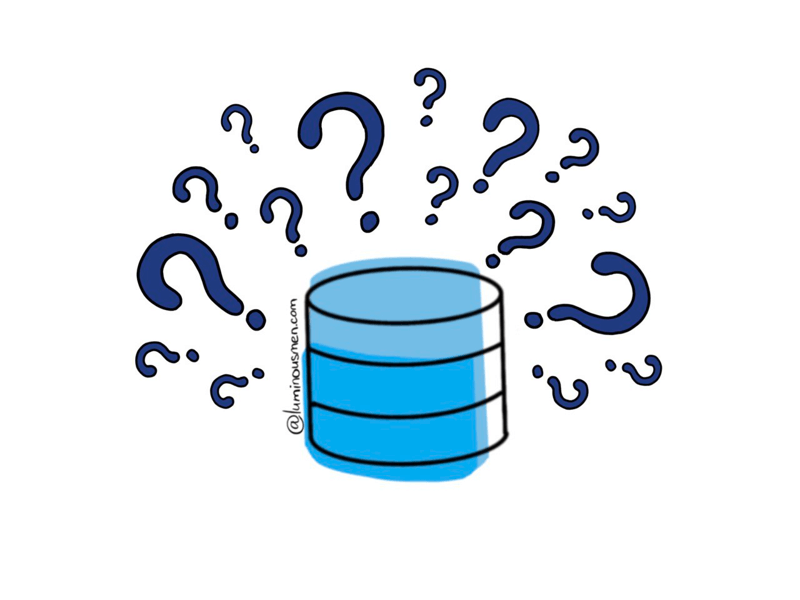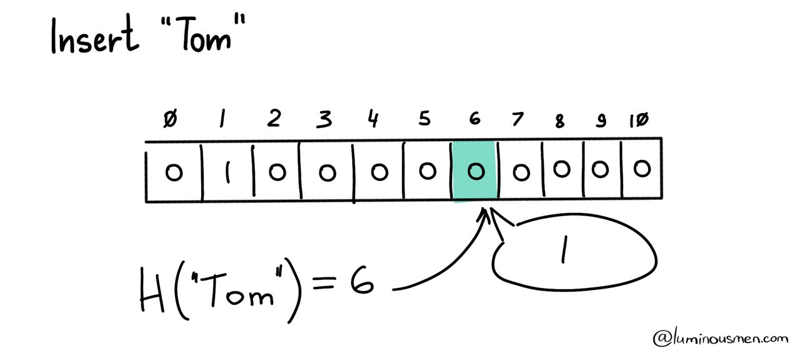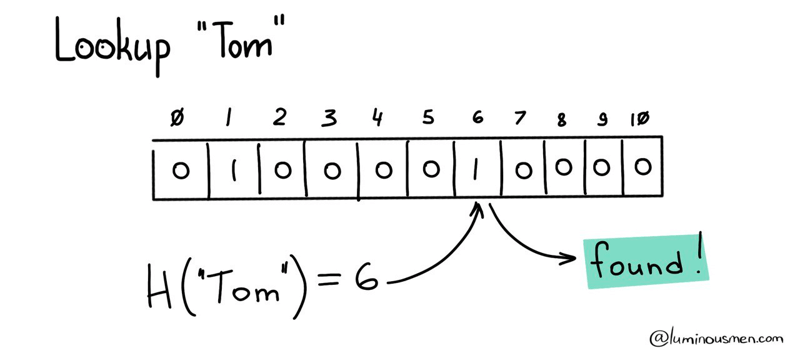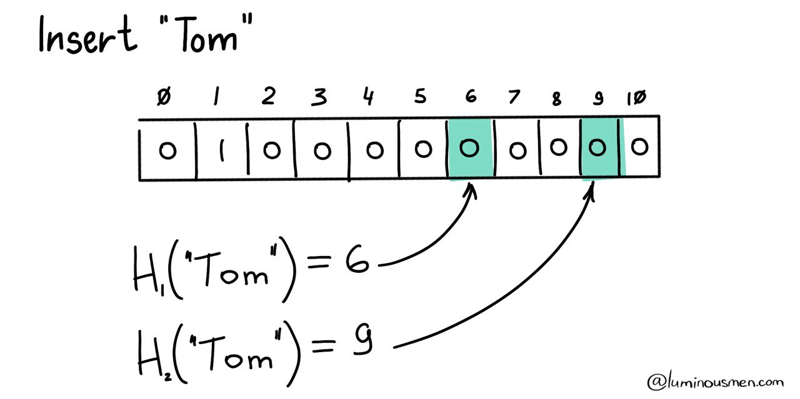Bloom filter: No means 'No', Yes means 'Maybe'.

In this post, we will explore the Bloom filter — a data structure that is ingenious in its simplicity and elegant in its design. We will delve into the underlying principles of Bloom filters, understand its benefits and drawbacks, and walk you through the process of implementing a Bloom filter using Python.

Bloom filters are a popular topic in computer science, particularly in systems design and optimization, and in technical interviews. Understanding this data structure and how to implement it can be an excellent way to showcase your skills as a data engineer.

## ProblemThe problem that Bloom filters aim to solve is straightforward — to determine if an element belongs to a dataset or not. This task becomes challenging when datasets are so large that they cannot fit into the memory of a single computer or even on a hard disk, especially in a distributed system where data is stored across multiple nodes. In such a scenario, the communication overhead associated with disk access makes the task even more challenging.

## A way to probabilistic data structure

Traditional approaches, such as iterating over the dataset and comparing each element can be very time consuming and impractical in large datasets (even if we use distributed frameworks such as MapReduce or Spark). We would prefer a solution that provides near real-time results.

One possible solution is to use indexes similar to those used in databases. These indexes contain sorted keys and pointers to the corresponding elements in the database, which allows us to quickly find an element by its key. However, in our case, we don't even need the actual value of the element; we just need to know whether a value exists for a given key or not. Storing actual values would be a waste of storage space.

If we don't need the actual value, we can compress the element with something to avoid wasting storage space. The next thing that comes to mind is the use of hash functions, that is, functions that map arbitrary size data to fixed size data. We simply calculate the hash value of each element and write some value to the array at given offset. The offset in this case is the remainder of the hash value divided by the array size. Or in pseudo code it would look something like this: `array[hash(element) % size] = 1` The logic is the same as regular hash tables, with the exception that we do not store chains of elements with the same hash value to save space.This approach compresses the search data significantly, making it ideal for our large dataset. To check if an element is in the dataset, we simply calculate the hash value for the potential member and check if the corresponding array value is not set to zero.

To check that the element is in the dataset, as you have already guessed, it is enough to calculate the hash value for the potential member and make sure that the corresponding array value is not set to zero.But there is one drawback here…

Hash functions work on a many-to-one basis, meaning that multiple elements can have the same hash value, but each element has only one unique hash. This can result in collisions, where multiple elements have the same hash value, and in turn lead to false positives when searching the array due to these collisions.

In this case, we accept the possibility of false positives without taking any additional action. It's more likely that an element doesn't exist in the dataset, even if that is a huge dataset, since not all possible elements are typically present. And our data structure is guaranteed to correctly determine that the element doesn't exist, because otherwise the hash function would produce the value we have. And that's how we come to the probabilistic nature of this data structure.

## More improvements

What do we have at the moment? In terms of speed, the algorithm will work just fine — at best we only count the hash function and take the offset value, but in terms of space, meh — probably not. Because if we want to minimize the probability of false positives, we need a big ass array.

To solve this problem, let's use bitmask, where we pick up the bit at the offset corresponding to the value of the hash function. No difference in terms of logic, but a huge gain in terms of memory usage.

To further minimize the probability of false positives, we will use several hash functions and raise several bits in our bitmask corresponding to their values. To check, we'll calculate the same hash functions and check the bits. If all the bits are raised — then most likely we have an element (or maybe not because of collisions). If at least one bit is not raised — then we definitely do not have it.With these improvements, we now have a Bloom filter.

## A bit of theory

A Bloom filter is a probabilistic data structure that is used to determine whether an element belongs to a set.

The basic idea is to use a fixed-size bit array and a set of hash functions to map elements from the dataset to the bit array. Each hash function maps an element to one or more positions in the bit array. When an element is added to the dataset, the corresponding bits in the bit array are set to 1. To check if the element is in the dataset, the same hash functions are used to map the element to the bit array, and the corresponding bits are checked. If all bits are 1, the element is probably in the dataset (with a certain probability of false positives). If any of the bits are 0, the element is definitely not in the dataset .The best part? Bloom filters guarantee that there will never be false negatives. Meaning, if the element is not in the set, the Bloom filter will never say it is present. Similarly, if the element is in the set, the Bloom filter will always correctly report that it is, and never mistakenly report that it is not. However, there may be false positives, where the Bloom filter may report that an element is in the set even though it is not. But don't worry, probability of false positives can be controlled by changing the size of the table used for the Bloom filter, as well as by varying the number of hash functions.

### Bloom filter operations

You can perform operations on sets using Bloom filters by creating multiple Bloom filters, one for each set you want to work on, and then applying bitwise operations on the filter bits.

For example, to perform an intersection of two sets, you can create a Bloom filter for each set and then perform a bitwise `AND` operation on the bits of the two filters. The resulting filter will have a 1 in the bit position only if that position was a 1 in both original filters. Similarly, to combine two sets (perform a union operation), you could create two Bloom filters for each set, and then perform a logical `OR` on the bits of the two filters. The resulting filter will have a 1 in the bit position if that position was a 1 in at least one of the original filters. But wait, there's a catch. The resulting filter will be probabilistic, meaning that there may be false positives in the result.

Here's the cherry on top: you can even estimate the size of the original dataset with a Bloom filter! All you need is the size of the filter and the number of raised bits. Stay tuned for the implementation details.

### Hash functions

Hash functions are a critical component of Bloom filters, and choosing the right one is essential for optimal performance. Instead of using cryptographic hash functions like MD5, which are slow, Bloom filters usually use faster non-cryptographic hash functions like MurmurHash or FNV.

But speed isn't the only factor to consider. Independence of hash functions is crucial to ensure accurate results. It refers to the property that the output of hash functions should be as uncorrelated as possible. If hash functions aren't independent, there's a high chance they'll produce the same result for a given input, leading to more collisions and false positives.

The solution? Use multiple hash functions to increase independence. Combining different algorithms or using different seeds can help ensure that the hash functions produce unique outputs, reducing the collision probability and increasing the accuracy of the filter.

## Pros and cons

### Space efficiency [PRO]

The main advantage of the Bloom filter is that it is very compact. Instead of storing the actual values, we only need to store the bitmask, which takes up much less space. For example, you can represent 10 million records, taking up about 40 MB of space. This makes it especially useful for large data sets that cannot fit in the memory of a single computer or even on a hard disk.

### Speed [PRO]

The Bloom filter is a lightning-fast tool for checking the existence of an element in a set. With just a few quick calculations of hash functions and a check of the corresponding bits in the bit array, you can determine if an element is present with a constant time and space complexity of O(k), where k is the number of hash functions. This means that even as the number of hash functions increases, the speed remains constant.

Plus, adding an element to the Bloom filter is just as quick, taking only O(k) constant time. And even if a search doesn't find the element on the first try, the well-tuned Bloom filter will often find it in just one or two additional trials, making the search operation just as speedy as the insertion operation.

### Scalability [PRO]

The construction of the Bloom filter can be effectively parallelized. This means that we can divide the original array into parts, build a separate filter on each of them in parallel, and then merge them. This not only helps to avoid a bottleneck in one place, for example on the Spark-driver, but also allows for parallel processing on multiple nodes.

### Probabilistic data structure [CON]

While the Bloom filter is a powerful tool, it's important to keep in mind its potential limitations. One key disadvantage is that it's a probabilistic data structure, which can result in false positives. This happens when the hash functions cause an element that's not in the set to be mistakenly recognized as if it were.

The likelihood of false positives can be reduced by using multiple hash functions and a larger bit array. Just keep in mind that doing so will increase the space requirements of your Bloom filter.

### Not support removing elements [CON]

Another drawback of the Bloom filter is that it does not support removing elements from the set. Once you add something to the set, it stays there forever.

This issue can be resolved by opting for a counting Bloom filter, which not only allows you to add elements to the set, but also gives you the power to remove them by reducing the corresponding bits in the bit array. So, you get the best of both worlds — the speed and efficiency of the Bloom filter, and the flexibility to manage your set as you see fit.

### Limited Resizing Capabilities [CON]

One of the downsides of Bloom filters is that they can be difficult to resize effectively. Unlike other data structures that store elements directly, Bloom filters don't keep a record of the elements they've seen. So, if you want to build a larger Bloom filter, you'll need to retrieve all of the original keys from permanent storage.

However, there is a solution to this challenge. Scalable Bloom filters, as the name implies, are Bloom filters that can automatically resize as the number of elements in the filter grows. This is achieved through the use of a series of smaller Bloom filters, each with a fixed size, that are combined into a chain. As the number of elements in the filter increases, more Bloom filters are added to the chain, effectively increasing its size.

## Implementation

Now that we have a solid understanding of how Bloom filters work, let's dive into the implementation. A basic Bloom filter is quite simple to implement. Here is a sample code in python:

``````import hashlib
import math

class BloomFilter:
def __init__(self, size, num_hashes, salt=None):
self.size = size
self.num_hashes = num_hashes
self.salt = salt or ''
self.bit_array =  * size

for i in range(self.num_hashes):
digest = hashlib.sha1((self.salt + str(element) + str(i)).encode('utf-8')).hexdigest()
index = int(digest, 16) % self.size
self.bit_array[index] = 1

def lookup(self, element):
for i in range(self.num_hashes):
digest = hashlib.sha1((self.salt + str(element) + str(i)).encode('utf-8')).hexdigest()
index = int(digest, 16) % self.size
if self.bit_array[index] == 0:
return False
return True

def estimate_dataset_size(self):
m = self.size
k = self.num_hashes
n = -(m / k) * math.log(1 - sum(self.bit_array) / m)
return n

def union(self, other):
if self.size != other.size or self.num_hashes != other.num_hashes:
raise ValueError("Both filters must have the same size and hash count")
result = BloomFilter(self.size, self.num_hashes)
result.bit_array = [x | y for x, y in zip(self.bit_array, other.bit_array)]
return result

def intersection(self, other):
if self.size != other.size or self.num_hashes != other.num_hashes:
raise ValueError("Both filters must have the same size and hash count")
result = BloomFilter(self.size, self.num_hashes)
result.bit_array = [x & y for x, y in zip(self.bit_array, other.bit_array)]
return result
``````

The number of hash functions used is specified by the `num_hashes` argument, and the size of the bit array is specified by the `size` argument. The `salt` argument allows for additional randomization of the hash values. The `add` method sets the bits corresponding to the hash values of an element, and the `__contains__` method checks if all the bits corresponding to the hash values of an element are set.

In this implementation, the `BloomFilter` class uses the SHA1 hash function to map elements to the bits in the bit array, all of which are initially set to 0. It is just the same hash function with different salt, the value added to the hashed value. In our implementation, the first hash function adds `0`, the second adds `1`, and so on.

For the sake of clarity, we've used an integer list here, but the real implementation will only take up one bit per element. Also for readability, example use size equals to 20. Typical real life Bloom filters have size that is about 9.6 times the expected number of elements in the set. This gives a false positive rate of about 1%.

Now, let’s test the code:

``````if __name__ == "__main__":
coffees = [
"Iced Coffee",
"Iced Coffee with Milk",
"Espresso",
"Espresso Macchiato",
"Flat White",
"Latte Macchiato",
"Cappuccino",
"Mocha",
]

bloom = BloomFilter(20, 2)
for drink in coffees:
print(bloom.bit_array)

print("---Experiment #1---")
print(bloom.lookup("Flat White"))  # True
print(bloom.lookup("Americano")) # True?! Wat?
print(bloom.estimate_dataset_size())  # 7.985076962177717

more_coffees = [
"Iced Espresso",
"Flat White",
"Cappuccino",
"Frappuccino",
"Latte",
]

bloom2 = BloomFilter(20, 2)
for drink in more_coffees:

bloom3 = bloom2.union(bloom)
print("---Experiment #2---")
print(bloom3.lookup("Mocha"))  # True
print(bloom3.lookup("Frappuccino"))  # True
print(bloom3.estimate_dataset_size())  # 13.862943611198906

bloom4 = bloom2.intersection(bloom)
print("---Experiment #3---")
print(bloom4.lookup("Mocha"))  # False
print(bloom4.lookup("Flat White"))  # True
print(bloom4.estimate_dataset_size())  # 3.5667494393873245
``````

## Usage

Networking: Bloom filters are used in distributed systems to check if a certain piece of data exists on a remote host without having to transfer the data. This is especially useful in situations where memory is limited, such as in network routers, network packet analysis tools, and internet caches.

Caching: Bloom filters are used to quickly check if some data is in the cache before retrieving it from the source. By using a Bloom filter, you can significantly reduce the load on your disk while only spending a small amount of RAM. For example, if you want to reduce the load by a factor of 10, you only need to store about 5 bits of information per key. And if you want to reduce it by a factor of 100, you need about 10 bits per key. The choice is yours! One well-known example of this use case is Cassandra.

Databases: Bloom filters are used to check if a record exists in a database before performing a more expensive operation, such as a full table scan. By using a Bloom filter, you can avoid costly operations and speed up your database queries.

Big Data: Bloom filters have become the go-to solution for systems that handle large data sets. Their efficiency in situations where you need the functionality of a hash table but don't have enough space makes them a popular choice for use in networks and distributed databases. For example, Google uses Bloom filters in its BigTable to reduce the number of disk accesses. This is because BigTable is essentially a large, very sparse multi-dimensional table, so most of the keys don't point to anything.

## Alternatives

Cuckoo Filter and Hyperloglog are two alternatives to the Bloom filter. They are both used for probabilistic set membership tests and have their own unique advantages and disadvantages compared to the Bloom Filter.

Let's start with the Cuckoo Filter. The naming is inspired by the cuckoo bird and its aggressive behavior in taking over other birds' nests. Just like the cuckoo bird, the Cuckoo Filter is known for its aggressive use of space and its ability to efficiently take over memory. This filter is designed to use less space compared to Bloom filters, but with a trade-off of a higher false positive rate.

Next, let's look at Hyperloglog. The name Hyperloglog is derived from the fact that it uses logarithms and logarithmic scaling. Unlike the Bloom filter and Cuckoo filter, the Hyperloglog is not designed to test set membership but to estimate the cardinality of a set. This means it can be used to count the number of unique elements in a set. The Hyperloglog is designed to be highly accurate, even with extremely large data sets, but with a trade-off of increased memory usage compared to Bloom filters. For example, Presto, the distributed SQL query engine, uses HyperLogLog to get an approximate number of individual items.

## Summary

In conclusion, Bloom filters are the unsung heroes of big data, offering a compact and efficient solution for checking membership in large datasets. Its clever design, which balances accuracy and speed, has made it a staple in various industries, from networking to distributed data systems. With the ability to improve system efficiency, it's no wonder why Bloom filters have become an essential tool for data engineers and computer science professionals alike.

But despite its strengths, the Bloom filter does have some limitations, such as the possibility of false positives and the inability to remove items from the set. However, these trade-offs are often outweighed by the benefits that the Bloom filter brings to the table.

### Materials

More? Well, there you go: